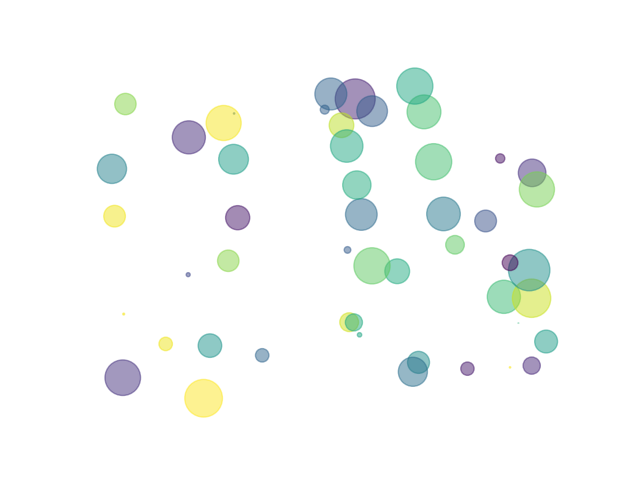# Python入门导读

很多培训机构宣称py是人工智能必备的编程语言，打着速成的旗号来引诱学者学习python。事实却并不是这样的，万丈高台平地起，不论你想从事怎样的编程工作，都是从最基本的编程技巧开始的；Python并不适合所有人，如果你是一个编程类专业的学生，适度了解python是有必要的（python的第三方库的爆发造就了不少C/C++程序员的就业），但如果你作为一个非编程类专业但又需要了解编程的人，我强烈推荐你学习python。

想要学习Python,你应该有一本Python的入门书，如果有一定的编程基础推荐使用《python cookbook》，第二步是在自己的电脑上安装python，我已经为你写好了安装指南，https://blog.csdn.net/m0_37772174/article/details/84867052。当有一定python基础时，一定要在官网[https://www.python.org]寻找解决方案，不论是哪种编程语言，官网的资料才是主食，其余任何作者写的Python编程内容都只能作为零食。

不应该过分推崇Python，也不要对Python存在偏见；编程语言存在一个先入原则，程序员总会对自己最擅长的编程语言有所偏爱，但经久而不衰的编程语言恐怕只有Java,C/C++了；笔者最擅长的语言是C++，但笔者仍然认为，未来最为火爆的编程语言一定是python。Python能让更多的人享受编程。

使用Python，短短几行代码就能让人画出不一样的图案

``````#时间:2019/7/26
#作者:cclplus
import numpy as np
import matplotlib.pyplot as plt

if __name__ =="__main__":
N = 50
x = np.zeros(N)
y = np.zeros(N)
for i in range(N):
x[i] = np.random.rand()
y[i] = np.random.rand()
colors = np.random.rand(N)
area = (30 * np.random.rand(N))**2  # 0 to 15 point radii
ax = plt.subplot(111)
ax.scatter(x, y, s=area, c=colors, alpha=0.5)

ax.spines['top'].set_visible(False)
ax.spines['right'].set_visible(False)
ax.spines['bottom'].set_visible(False)
ax.spines['left'].set_visible(False)
plt.axis('off')
plt.savefig('scatter.png', transparent=True)``````

执行这段代码后，在该文件的目录下就能找到生成的图片，可以用这种方法为自己的文章生成封面，因为气泡的颜色和大小以及位置都是用随机数来确定，基本不用担心会出现一样的封面。如果你对编程感兴趣，你可以学习数据结构，因为在设计程序时，数据结构实在是太重要了。很多看似简单的程序，如果没有很好的数据结构的功底往往会无从下手。Python不仅适用于编程入门新手，Python同样适用于成熟的程序员，对一个从事机器视觉软件开发的程序员来说，毫无疑问C++是机器视觉软件开发者最应该掌握的编程语言，其次就应该是Python。

以下是一段广告，诚信可靠：欢迎购买该课程入门python，特惠期只要CN￥:99

https://study.163.com/course/courseMain.htm?courseId=1005699008&share=2&shareId=400000000537035

• 微信
• 扫描二维码添加微信
•• 博客交流群
• 来这里水过美好的一天
•